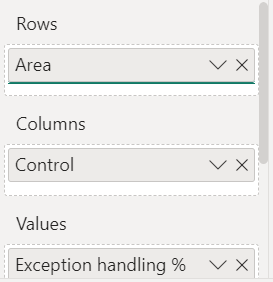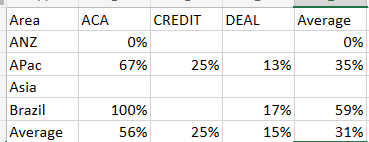cancel
Showing results for
Did you mean:Microsoft

## Need to display average instead of totals in a matrix

Hi Team,

As you can see i have a matrix visualization here , Having Rows : Area , Columns : Controls , And Values : A measure

i need to find the average but the average is showing here as wrong as below picture :Data given in rows,columns and values :My expected outcome is :Example for averages : ACA average is calculated as 0/1=0%

Brazil Average is calculated as 100+17/2=59%

1 ACCEPTED SOLUTIONSuper User

Then use

``````Exception HAndling % Average Total =
AVERAGEX (
SUMMARIZE ( 'Table', 'Table'[Area], 'Table'[Control] ),
[Exception HAndling %]
)``````
6 REPLIES 6Super User

Please try this measure in the values of the matrix

``````Exception HAndling % Average Total =
AVERAGEX ( VALUES ( 'Table'[Control] ), [Exception HAndling %] )``````Microsoft

Hey thanks for the quick reply,

now the row wise average are correct

but column wise is wrongSuper User

Are area and Control from the same table? If different tables, would you please provide details about the relationships?Microsoft

coming from same tableSuper User

Then use

``````Exception HAndling % Average Total =
AVERAGEX (
SUMMARIZE ( 'Table', 'Table'[Area], 'Table'[Control] ),
[Exception HAndling %]
)``````Microsoft

its working now thank you